# 一、跳表的基本概念

### 2、跳表的详解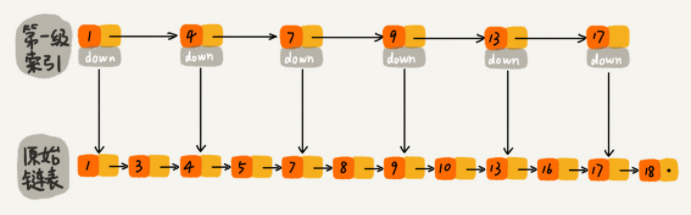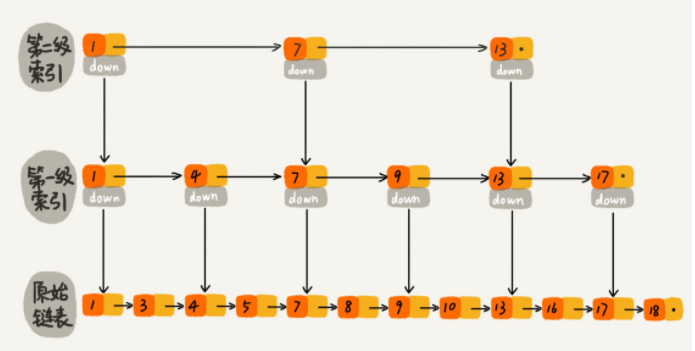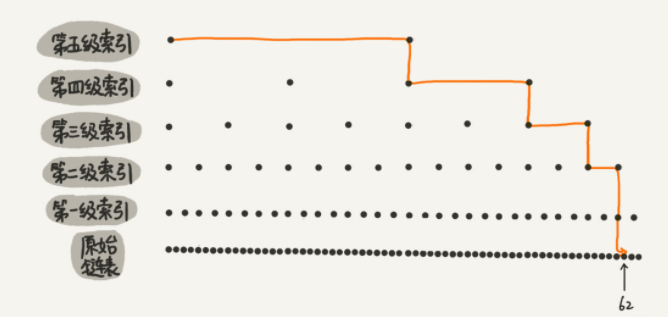### 3、跳表的时间复杂度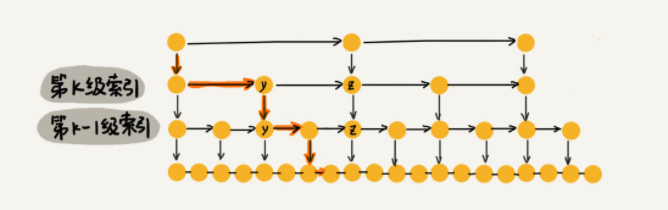### 4、跳表的空间复杂度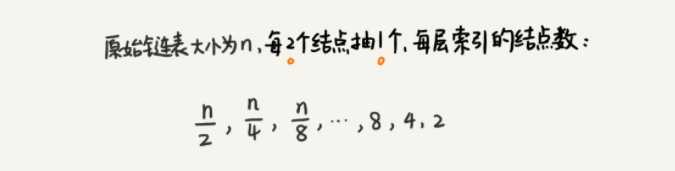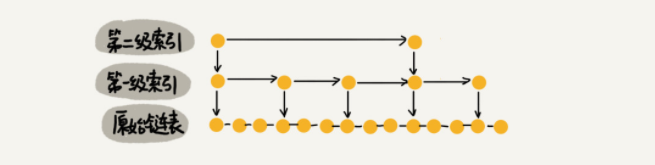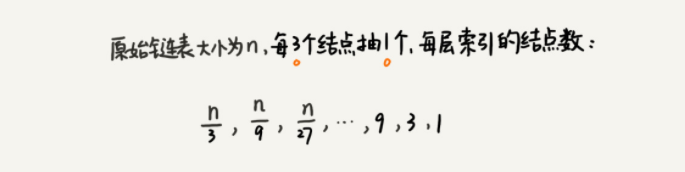### 5、跳表的插入### 7、跳表索引动态更新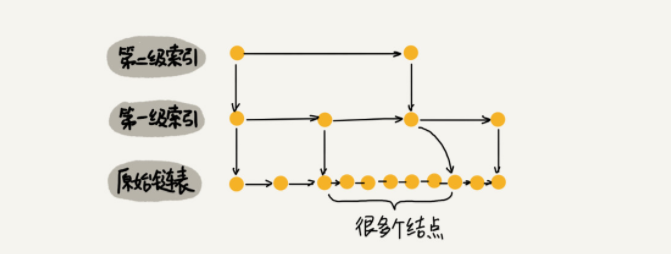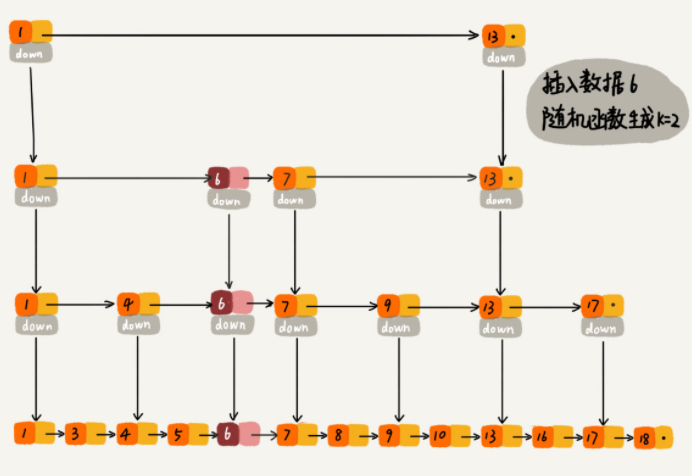### 8、跳表的性质

(1) 由很多层结构组成，level是通过一定的概率随机产生的；
(2) 每一层都是一个有序的链表，默认是升序 ；
(3) 最底层(Level 1)的链表包含所有元素；
(4) 如果一个元素出现在Level i 的链表中，则它在Level i 之下的链表也都会出现；
(5) 每个节点包含两个指针，一个指向同一链表中的下一个元素，一个指向下面一层的元素。

# 二、跳表的Java代码实现

// 跳表中存储的是正整数，并且存储的数据是不重复的
public class SkipList {

private static final int MAX_LEVEL = 16;    // 结点的个数

private int levelCount = 1;   // 索引的层级数

private Node head = new Node();    // 头结点

private Random random = new Random();

// 查找操作
public Node find(int value){
for(int i = levelCount - 1; i >= 0; --i){
while(p.next[i] != null && p.next[i].data < value){
p = p.next[i];
}
}

if(p.next != null && p.next.data == value){
return p.next;    // 找到，则返回原始链表中的结点
}else{
return null;
}
}

// 插入操作
public void insert(int value){
int level = randomLevel();
Node newNode = new Node();
newNode.data = value;
newNode.maxLevel = level;   // 通过随机函数改变索引层的结点布置
Node update[] = new Node[level];
for(int i = 0; i < level; ++i){
}

for(int i = level - 1; i >= 0; --i){
while(p.next[i] != null && p.next[i].data < value){
p = p.next[i];
}
update[i] = p;
}

for(int i = 0; i < level; ++i){
newNode.next[i] = update[i].next[i];
update[i].next[i] = newNode;
}
if(levelCount < level){
levelCount = level;
}
}

// 删除操作
public void delete(int value){
Node[] update = new Node[levelCount];
for(int i = levelCount - 1; i >= 0; --i){
while(p.next[i] != null && p.next[i].data < value){
p = p.next[i];
}
update[i] = p;
}

if(p.next != null && p.next.data == value){
for(int i = levelCount - 1; i >= 0; --i){
if(update[i].next[i] != null && update[i].next[i].data == value){
update[i].next[i] = update[i].next[i].next[i];
}
}
}
}

// 随机函数
private int randomLevel(){
int level = 1;
for(int i = 1; i < MAX_LEVEL; ++i){
if(random.nextInt() % 2 == 1){
level++;
}
}

return level;
}

// Node内部类
public class Node{
private int data = -1;
private Node next[] = new Node[MAX_LEVEL];
private int maxLevel = 0;

// 重写toString方法
@Override
public String toString(){
StringBuilder builder = new StringBuilder();
builder.append("{data:");
builder.append(data);
builder.append("; leves: ");
builder.append(maxLevel);
builder.append(" }");
return builder.toString();
}
}

// 显示跳表中的结点
public void display(){
while(p.next != null){
System.out.println(p.next + " ");
p = p.next;
}
System.out.println();
}

}


（1）Redis中的有序集合是通过跳表来实现的，当然还用到了散列表。

（2）大部分编程语言中的Map类型都是通过红黑树实现的，我们在写程序的时候，可以直接拿过来用，不用自己再去实现一个红黑树。但是跳表并没有一个现成的实现，所以在开发中，如果要使用跳表这种数据结构，需要自己先去实现。

## 参考及推荐：

1、跳表Java实现

2、Java基础 - 跳表（SkipList）

05-2763504-25882
03-171万+
12-26372
12-184万+
03-11105
03-015214
09-309525